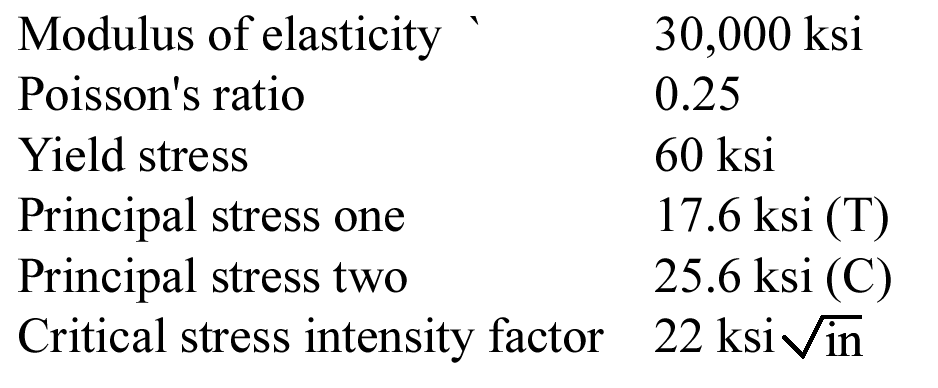# 2.2 – MATERIAL MODELS

Using the information below, choose the correct answer to the closest 3 significant figures. For questions 1 through 5, assume plane stress. For questions 6 through 10, assume plane strain.Name Email
1. Assume plane stress:
The principal strain one is
2. Assume plane stress:
The principal strain three is
3. Assume plane stress:
The factor of safety to avoid yielding using maximum shear stress theory is
4. Assume plane stress:
The factor of safety to avoid yielding using maximum octahedral shear stress theory is
5. Assume plane stress:
The critical crack length in Mode I is
6. Assume plane strain:
The principal stress three is
7. Assume plane strain
The principal strain one is
8. Assume plain strain:
The factor of safety to avoid yielding using maximum shear stress theory is
9. Assume plane strain:
The von-Mises stress is
10. Assume plane strain:
The factor of safety to avoid yielding using maximum octahedral shear stress theory is